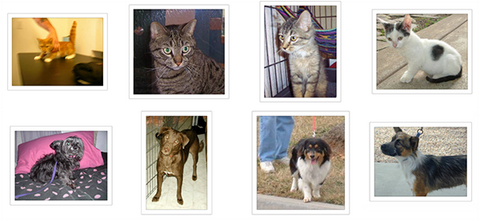您当前的位置：首页 > IT编程 > 数据集 | C语言 | Java | VB | VC | python | Android | TensorFlow | C++ | oracle | 学术与代码 | cnn卷积神经网络 | gnn | 图像修复 | Keras | 数据集 | Neo4j | 自然语言处理 | 深度学习 | 医学CAD | 医学影像 | 超参数 | pointnet | pytorch |

# 小样本数据集图像分类模型的方法

51自学网 2020-12-08 10:04:49
数据集

• 从头开始训练小型网络（作为基线）
• 使用预先训练的网络的瓶颈功能
• 微调预训练网络的top layers

• `fit_generator` 使用Python数据生成器，训练Keras模型
• `ImageDataGenerator` 用于实时数据增强
• 层冻结（layer freezing）和模型fine-tuning

## 开工：2000个训练样本（每类1000个）

• 安装了Keras，SciPy，PIL的电脑(当然如果有Nvidia 的GPU最好了)。
• 训练数据集和验证数据集，目录如下：
``````data/
train/
dog/
dog001.jpg
dog002.jpg
...
cats /
cat001.jpg
cat002.jpg
...
validation /
dogs /
dog001.jpg
dog002.jpg
...
cats /
cat001.jpg
cat002.jpg
...``````

“在多年前进行的非正式民意调查中，计算机视觉专家认为，如果没有现有技术的重大进步，精度高于60％的分类器将很难实现。目前的文献表明，机器分类器在此任务上的准确度可以达到80％以上[参考]。“

## 数据预处理和数据增强

• 配置训练过程中图像的各种变换和归一化操作
• 通过调用`.flow(data, labels)`或者`.flow_from_directory(directory)`方法，返回上述操作的图像batch生成器。这个生成器可以和Keras的模型方法（`fit_generator`,`evaluate_generator和predict_generator`）一起使用，作为他们的输入。

``````from keras.preprocessing.image  import ImageDataGenerator

datagen  =  ImageDataGenerator （
rotation_range = 40 ，
width_shift_range = 0.2 ，
height_shift_range = 0.2 ，
rescale= 1 / 255 ，
shear_range = 0.2 ，
zoom_range = 0.2 ，
horizontal_flip = True，
fill_mode = 'nearest' ）``````

• `rotation_range` 是一个度数（0-180）的值，表示随机旋转图片的范围
• `width_shift和height_shift`是在垂直或水平方向上随机平移图片的范围（作为总宽度或高度的一部分）
• `rescale`是一个值，对图像的亮度值进行缩放，这个操作在所有操作之前，例如rescale为1/255时，表示把RGB的值从0-255转换到0-1之间。
• `shear_range`用于随机应用剪切变换
• `zoom_range` 用于随机缩放
• `horizontal_flip` 50%的随机概率对图像进行水平翻转
• `fill_mode` 像素填充策略，在进行旋转或平移之后，需要对图像像素进行填充。

``````from keras.preprocessing.image import ImageDataGenerator, array_to_img, img_to_array, load_img

datagen = ImageDataGenerator(
rotation_range=40,
width_shift_range=0.2,
height_shift_range=0.2,
shear_range=0.2,
zoom_range=0.2,
horizontal_flip=True,
fill_mode='nearest')

img = load_img('data/train/cats/cat.0.jpg')  # this is a PIL image
x = img_to_array(img)  # this is a Numpy array with shape (3, 150, 150)
x = x.reshape((1,) + x.shape)  # this is a Numpy array with shape (1, 3, 150, 150)

# the .flow() command below generates batches of randomly transformed images
# and saves the results to the `preview/` directory
i = 0
for batch in datagen.flow(x, batch_size=1,
save_to_dir='preview', save_prefix='cat', save_format='jpeg'):
i += 1
if i > 20:
break  # otherwise the generator would loop indefinitely``````

## 从头开始训练一个小卷积神经网络：40行代码，准确率达到80％

``````from keras.models import Sequential
from keras.layers import Conv2D, MaxPooling2D
from keras.layers import Activation, Dropout, Flatten, Dense

model = Sequential()
model.add(Conv2D(32, (3, 3), input_shape=(3, 150, 150)))

# the model so far outputs 3D feature maps (height, width, features)``````

``````model.add(Flatten())  # this converts our 3D feature maps to 1D feature vectors

model.compile(loss='binary_crossentropy',
optimizer='rmsprop',
metrics=['accuracy'])``````

``````batch_size = 16

# this is the augmentation configuration we will use for training
train_datagen = ImageDataGenerator(
rescale=1./255,
shear_range=0.2,
zoom_range=0.2,
horizontal_flip=True)

# this is the augmentation configuration we will use for testing:
# only rescaling
test_datagen = ImageDataGenerator(rescale=1./255)

# this is a generator that will read pictures found in
# subfolers of 'data/train', and indefinitely generate
# batches of augmented image data
train_generator = train_datagen.flow_from_directory(
'data/train',  # this is the target directory
target_size=(150, 150),  # all images will be resized to 150x150
batch_size=batch_size,
class_mode='binary')  # since we use binary_crossentropy loss, we need binary labels

# this is a similar generator, for validation data
validation_generator = test_datagen.flow_from_directory(
'data/validation',
target_size=(150, 150),
batch_size=batch_size,
class_mode='binary')``````

``````model.fit_generator(
train_generator,
steps_per_epoch=2000 // batch_size,
epochs=50,
validation_data=validation_generator,
validation_steps=800 // batch_size)
model.save_weights('first_try.h5')  # always save your weights after training or during training``````

## 使用预先训练的网络的瓶颈功能：一分钟内准确率达到90％

``````batch_size = 16

generator = datagen.flow_from_directory(
'data/train',
target_size=(150, 150),
batch_size=batch_size,
class_mode=None,  # this means our generator will only yield batches of data, no labels
shuffle=False)  # our data will be in order, so all first 1000 images will be cats, then 1000 dogs
# the predict_generator method returns the output of a model, given
# a generator that yields batches of numpy data
bottleneck_features_train = model.predict_generator(generator, 2000)
# save the output as a Numpy array
np.save(open('bottleneck_features_train.npy', 'w'), bottleneck_features_train)

generator = datagen.flow_from_directory(
'data/validation',
target_size=(150, 150),
batch_size=batch_size,
class_mode=None,
shuffle=False)
bottleneck_features_validation = model.predict_generator(generator, 800)
np.save(open('bottleneck_features_validation.npy', 'w'), bottleneck_features_validation)``````

``````train_data = np.load(open('bottleneck_features_train.npy'))
# the features were saved in order, so recreating the labels is easy
train_labels = np.array( * 1000 +  * 1000)

validation_labels = np.array( * 400 +  * 400)

model = Sequential()

model.compile(optimizer='rmsprop',
loss='binary_crossentropy',
metrics=['accuracy'])

model.fit(train_data, train_labels,
epochs=50,
batch_size=batch_size,
validation_data=(validation_data, validation_labels))
model.save_weights('bottleneck_fc_model.h5')``````

``````Train on 2000 samples, validate on 800 samples
Epoch 1/50
2000/2000 [==============================] - 1s - loss: 0.8932 - acc: 0.7345 - val_loss: 0.2664 - val_acc: 0.8862
Epoch 2/50
2000/2000 [==============================] - 1s - loss: 0.3556 - acc: 0.8460 - val_loss: 0.4704 - val_acc: 0.7725
...
Epoch 47/50
2000/2000 [==============================] - 1s - loss: 0.0063 - acc: 0.9990 - val_loss: 0.8230 - val_acc: 0.9125
Epoch 48/50
2000/2000 [==============================] - 1s - loss: 0.0144 - acc: 0.9960 - val_loss: 0.8204 - val_acc: 0.9075
Epoch 49/50
2000/2000 [==============================] - 1s - loss: 0.0102 - acc: 0.9960 - val_loss: 0.8334 - val_acc: 0.9038
Epoch 50/50
2000/2000 [==============================] - 1s - loss: 0.0040 - acc: 0.9985 - val_loss: 0.8556 - val_acc: 0.9075``````

## 微调预训练网络的top layers

• 实例化VGG16卷积网络，并加载预训练权重
• 在顶部添加我们先前定义的完全连接模型，并加载其权重
• 冻结VGG16模型的层到最后一个卷积块

• 为了进行微调，所有层都应该从训练有素的权重开始：例如，你不应该在预先训练好的卷积基础上打一个随机初始化的全连接网络。这是因为由随机初始化的权重触发的大梯度更新将破坏卷积基础中的学习权重。在我们的例子中，这就是为什么我们首先训练顶级分类器，然后才开始微调卷积权重。
• 我们选择仅微调最后的卷积块而不是整个网络以防止过度拟合，因为整个网络将具有非常大的熵容量并因此具有过度拟合的强烈倾向。低级卷积块学习的特征比较高级的卷积块更加通用，不那么抽象，所以保持前几个块固定（更一般的特征）并且只调整最后一个块（更专业的特征）是明智的 ）。
• 微调应该以非常慢的学习速率完成，通常使用SGD优化器而不是适应性学习速率优化器，例如RMSProp。这是为了确保更新的幅度保持非常小，以免破坏以前学过的功能。

``````# build a classifier model to put on top of the convolutional model
top_model = Sequential()

# classifier, including the top classifier,
# in order to successfully do fine-tuning

# add the model on top of the convolutional base

``````# set the first 25 layers (up to the last conv block)
# to non-trainable (weights will not be updated)
for layer in model.layers[:25]:
layer.trainable = False

# compile the model with a SGD/momentum optimizer
# and a very slow learning rate.
model.compile(loss='binary_crossentropy',
optimizer=optimizers.SGD(lr=1e-4, momentum=0.9),
metrics=['accuracy'])``````

``````batch_size = 16

# prepare data augmentation configuration
train_datagen = ImageDataGenerator(
rescale=1./255,
shear_range=0.2,
zoom_range=0.2,
horizontal_flip=True)

test_datagen = ImageDataGenerator(rescale=1./255)

train_generator = train_datagen.flow_from_directory(
train_data_dir,
target_size=(img_height, img_width),
batch_size=batch_size,
class_mode='binary')

validation_generator = test_datagen.flow_from_directory(
validation_data_dir,
target_size=(img_height, img_width),
batch_size=batch_size,
class_mode='binary')

# fine-tune the model
model.fit_generator(
train_generator,
steps_per_epoch=nb_train_samples // batch_size,
epochs=epochs,
validation_data=validation_generator,
validation_steps=nb_validation_samples // batch_size)``````

• 更具侵略性的数据扩充
• 更积极的辍学
• 使用L1和L2正则化（也称为“重量衰减”）
• 微调一个卷积块（同时更大的正则化）

Building powerful image classification models using very little data
blog.keras.io